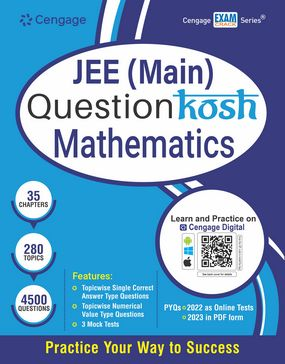###### Home
>

Test Prep### JEE (Main) QuestionKosh Mathematics

Author(s): Cengage India

ISBN: 9789355736147

Edition: 1st

#### Rs. 975

Binding: Paperback

Pages: 816

Trim Size : 279 x 216 mm

JEE (Main) QuestionKosh comprises a massive bank of exercises, which is set to practice subject concepts on a day-to-day basis. In order to synchronize aspirants’ preparation with their classroom lectures, the content lineup has been majorly kept parallel with that given in the NCERT book.

Book Features:

• Topicwise Single Correct Answer Type Questions
• Topicwise Numerical Value Type Questions
• 3 Mock Tests to help track your progress

Cengage Digital App Features

• PYQs 2022 as Online Tests
• PYQs 2023 in PDF form

1. Inequalities, Modulus and Logarithm
2. Sets
3. Relations and Functions I
5. Complex Numbers
6. Sequence and Series
7. Permutation and Combination
8. Binomial Theorem
9. Probability I
10. Trigonometric Functions
11. Trigonometric Equations
12. Solutions of Triangle
13. Straight Lines
14. Circle
15. Parabola
16. Ellipse and Hyperbola
17. Play with Graphs
18. Principle of Mathematical Induction
19. Statistics
20. Mathematical Reasoning
21. Introduction to Three Dimensional Geometry
22. Relations and Functions II
23. Inverse Trigonometric Functions
24. Matrices
25. Determinants
26. Limits, Continuity and Differentiability
27. Methods of Differentiation
28. Application of Derivatives
29. Indefinite Integrals
30. Definite Integrals
31. Differential Equations
32. Vector Algebra
33. Three Dimensional Geometry
34. Probability II
35. Linear Programming

Mock Test 1

Mock Test 2

Mock Test 3

Solutions